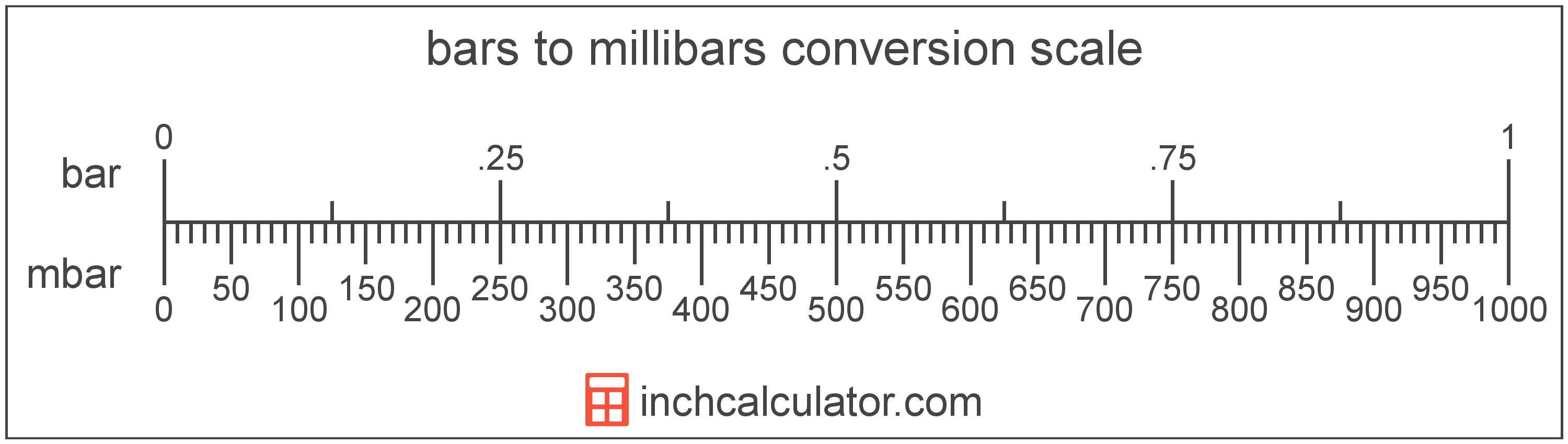# Millibars to Bars Converter

Enter the pressure in millibars below to get the value converted to bars.

Results in Bars:1 mbar = 0.001 bar

Do you want to convert bars to millibars?

## How to Convert Millibars to Bars

To convert a measurement in millibars to a measurement in bars, divide the pressure by the following conversion ratio: 1,000 millibars/bar.

Since one bar is equal to 1,000 millibars, you can use this simple formula to convert:

bars = millibars ÷ 1,000

The pressure in bars is equal to the pressure in millibars divided by 1,000.

For example, here's how to convert 500 millibars to bars using the formula above.
bars = (500 mbar ÷ 1,000) = 0.5 bar## What is a Millibar?

One millibar is equal to one-thousandth of a bar, which is defined as the pressure of one newton per square meter. One millibar is also equal to one hectopascal.

The millibar is a non-SI metric unit for pressure. In the metric system, "milli" is the prefix for thousandths, or 10-3. Millibars can be abbreviated as mbar; for example, 1 millibar can be written as 1 mbar.

## What is a Bar?

The bar is equal to 100,000 pascals, which are defined as the pressure of one newton per square meter. One bar is just less than the average atmospheric pressure.

The bar is a non-SI metric unit for pressure. Bars can be abbreviated as bar; for example, 1 bar can be written as 1 bar.

## Millibar to Bar Conversion Table

Table showing various millibar measurements converted to bars.
Millibars Bars
1 mbar 0.001 bar
2 mbar 0.002 bar
3 mbar 0.003 bar
4 mbar 0.004 bar
5 mbar 0.005 bar
6 mbar 0.006 bar
7 mbar 0.007 bar
8 mbar 0.008 bar
9 mbar 0.009 bar
10 mbar 0.01 bar
20 mbar 0.02 bar
30 mbar 0.03 bar
40 mbar 0.04 bar
50 mbar 0.05 bar
60 mbar 0.06 bar
70 mbar 0.07 bar
80 mbar 0.08 bar
90 mbar 0.09 bar
100 mbar 0.1 bar
200 mbar 0.2 bar
300 mbar 0.3 bar
400 mbar 0.4 bar
500 mbar 0.5 bar
600 mbar 0.6 bar
700 mbar 0.7 bar
800 mbar 0.8 bar
900 mbar 0.9 bar
1,000 mbar 1 bar